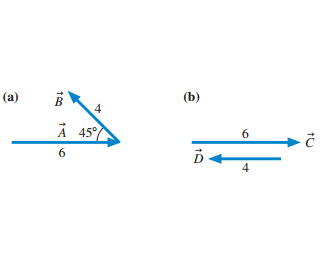# Problem: Evaluate the cross products A×B and C×D? Magnitude and Direction.

###### FREE Expert Solution

Cross-product:

$\overline{)\stackrel{\mathbf{⇀}}{\mathbf{A}}{\mathbf{×}}\stackrel{\mathbf{⇀}}{\mathbf{B}}{\mathbf{=}}{\mathbf{A}}{\mathbf{B}}{\mathbf{s}}{\mathbf{i}}{\mathbf{n}}{\mathbf{\theta }}}$###### Problem Details

Evaluate the cross products A×B and C×D? Magnitude and Direction.